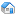Home ViewThis pageNormal viewPrintable viewExport to Adobe Acrobat (PDF)Export to Microsoft WordOther pagesHome pageDashboardNewsRecent updatesRSS feed builderIndexSite mapLabelsAttachments indexPeople DirectoryAccountLog inHistory Tools Primary Actions Secondary Modify Actions Browse Anonymous Anonymous User Actions

# Goals of Chombo Embedded Boundary Infrastructure

### Motivation

The goal of this software support is to provide a relatively compact set of abstractions in which the Cartesian grid embedded boundary algorithms we are developing can be expressed and implemented. The particular design we are proposing here is motivated by the following observations.

1. The dependent variables in a finite difference method are represented as arrays defined on subsets of an index space.
2. The transformations on arrays can be expressed as combinations of pointwise operations on the arrays, and of sums over nearby points of arrays, i.e., stencil operations.
3. For standard finite difference methods on rectangular grids, the index space is the D-dimensional rectangular lattice of D-tuples of integers, where D is the spatial dimension of the problem.
4. For multigrid or AMR methods, the index space is the hierarchy of \$d\$-dimensional rectangular lattices, where the successive members of the hierarchy are related to one another by coarsening and refinement operations. In both of these cases, the stencil operations can be expressed formally as a loop over stencil locations. In the AMR case, both the stencil locations and the locations where the stencil operations are applied are computed using a set calculus on the index space. If one fully exploits this picture to derive a set of abstractions for expressing these algorithms, it leads to a very concise implementation of the algorithms in these two domains.
5. The above characterization of finite difference methods holds for the EB algorithms as well, with the critical difference that the index space is no longer a rectangular lattice, but a more complicated object. In the case of a non-hierarchical grid representation, the index space is a combination of a rectangular lattice (the Cartesian grid part) and a graph representing the irregular cell fragments that abut the irregular boundary. For a hierarchical method, we have one such index space for each level of refinement, related to one another by coarsening and refinement operations. In addition, we want to support the overall implementation strategy that the bulk of the calculations (corresponding to data defined on the rectangular lattice) are performed using rectangular array representations, thus restricting the irregular array accesses and computations to a set of codimension one.

Overview of the EBChombo API
Here is a chart of the basic concepts in EBChombo. There are very important things left out but this gives the basic conceptual framework of the API.

Concept

Chombo

EBChombo

Z^D

(implied)

EBIndexSpace

Point in Z^D (node)

IntVect

VolIndex

Connection between points (edge)

(implied)

FaceIndex

Region over Z^D

Box

EBISBox

Union of Rectangles in Z^D

BoxLayout

EBISLayout

Data over region Z^D\$

BaseFab

BaseEBCellFAB, BaseEBFaceFAB

Iterator over points

BoxIterator

VoFIterator, FaceIterator

#### Search and Navigate Chombo:Chombo - Software for Adaptive Solutions of Partial Differential Equations

• No labels